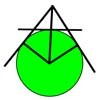#### You may also like### Mean Geometrically

A and B are two points on a circle centre O. Tangents at A and B cut at C. CO cuts the circle at D. What is the relationship between areas of ADBO, ABO and ACBO?### Without Calculus

Given that u>0 and v>0 find the smallest possible value of 1/u + 1/v given that u + v = 5 by different methods.### Pythagorean Golden Means

Show that the arithmetic mean, geometric mean and harmonic mean of a and b can be the lengths of the sides of a right-angles triangle if and only if a = bx^3, where x is the Golden Ratio.

# Three Ways

##### Age 16 to 18Challenge Level

Given that $x + y = -1$ find the largest value of $xy$

1. by coordinate geometry.
2. by calculus.
3. by algebra.